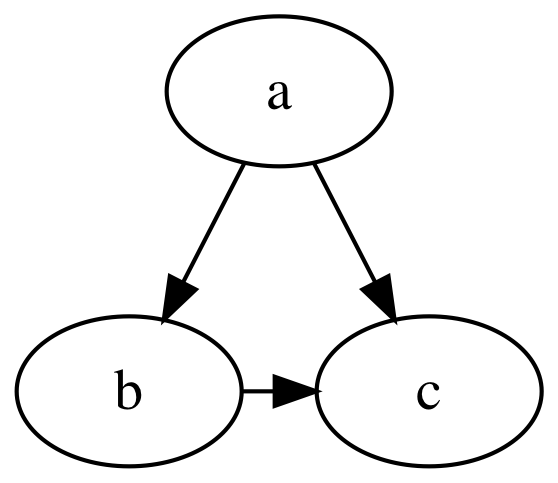## GraphViz

A Swift package for working with GraphViz.

• Swift 5.2+
• GraphViz

## Usage

``````import GraphViz

var graph = Graph(directed: true)

let a = Node("a"), b = Node("b"), c = Node("c")

graph.append(Edge(from: a, to: b))
graph.append(Edge(from: a, to: c))

var b_c = Edge(from: b, to: c)
b_c.constraint = false
graph.append(b_c)

// Render image to SVG using dot layout algorithm
graph.render(using: .dot, to: .svg) { result in
guard .success(let data) = result,
let svg = String(data: data, encoding: .utf8)
else { return }

print(svg)
}
````````````digraph {
a -> b
a -> c
b -> c [constraint=false]
}
``````

Note:
`render(using:to:)` and related methods require
GraphViz to be installed on your system.

### Using Function Builders, Custom Operators, and Fluent Attribute Setters

``````import GraphViz

let graph = Graph(directed: true) {
"a" --> "b"
"a" --> "c"
("b" --> "c").constraint(false)
}
``````

Note:
Swift 5.1 may require explicit typecast expressions in order to
reconcile use of custom edge operators like `-->`.
(`error: ambiguous reference to member '-->'`)

## Installation

### System Dependencies

You can install GraphViz on your system by running the following command:

``````# macOS
\$ brew install graphviz

# Linux (Ubuntu)
\$ sudo apt-get install graphviz
``````

Important:
and installed system dependencies using Homebrew,
Xcode may emit an error message like the following:

``````Warning: Could not load "/usr/lib/graphviz/libgvplugin_gdk.so.6"
It was found, so perhaps one of its dependents was not. Try ldd.
``````

One solution is to run the following commands to sign the dependencies
(replacing `MyName (MyTeam)` with your developer account name and team name):

``````\$ codesign -f -s "Apple Development: MyName (MyTeam)" /usr/local/opt/*/lib/*.dylib
\$ codesign -f -s "Apple Development: MyName (MyTeam)" /usr/local/Cellar/*/*/lib/*.dylib
``````

### Swift Package Manager

Add the GraphViz package to your target dependencies in `Package.swift`:

``````import PackageDescription

let package = Package(
name: "YourProject",
dependencies: [
.package(
url: "https://github.com/SwiftDocOrg/GraphViz",
from: "0.4.0"
),
]
)
``````

Add `GraphViz` as a dependency to your target(s):

``````targets: [
.target(
name: "YourTarget",
dependencies: ["GraphViz"]),
``````

## GitHub

https://github.com/SwiftDocOrg/GraphViz